Refer to our Texas Go Math Grade 1 Answer Key Pdf to score good marks in the exams. Test yourself by practicing the problems from Texas Go Math Grade 1 Lesson 12.1 Answer Key Add in Any Order.

Explore

Use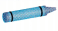and. Color to model the problem. Write the addition sentence.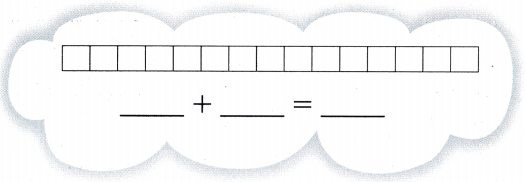Addition means summing up two or more numbers or values to get another number. For example, if we add 8 and 7, we get 15 as the result.
8+7=15
Now, let us understand the sum of 8 and 7 practically. Suppose, we have 8 apples in a basket and 7 more apples are added in the same basket, so how many apples are there altogether?
8: 1+1+1+1+1+1+1+1
7: 1+1+1+1+1+1+1
8+7=1+1+1+1+1+1+1+1+1+1+1+1+1+1+1.
Now if we count each apple, then it is equal to 5. The property of addition is to increase the value by adding another value to it.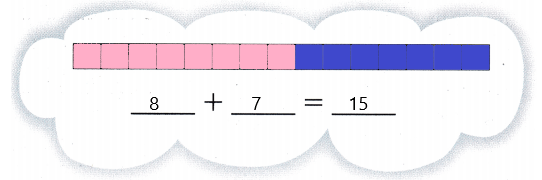Useand. Color to change the order. Write the addition sentence.By switching the numbers and adding seven with eight we get fifteen.

FOR THE TEACHER • Read the problem. George sees 7 blue birds and 8 red birds. How many birds does he see? Help children work through changing the order of the addends.
Explanation:
Given,
George sees seven blue birds and eight red birds,
By adding seven with eight we get fifteen,
By changing the order of addends,
We can switch numbers and by adding eight with seven we get fifteen,

Math Talk
Mathematical Processes

Explain how knowing the fact 7 + 8 helps you find 8 + 7.
By adding seven with eight we get fifteen,
By changing the order of the addends, the sum will not change it remains the same,
In the same way we can add eight with seven to get fifteen.

Model and Draw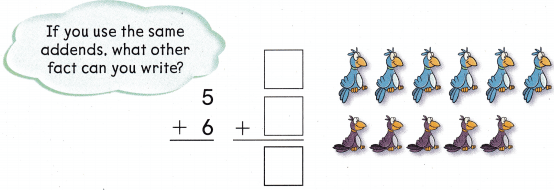Given,
By adding five with six we get eleven,
In the same way by changing the order of addends,
We can add six with five to get eleven,
Sum of the addends will not change even though the order of addends changes.

Share and Show

Question 1.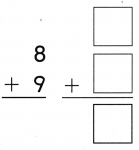Given,
By adding eight with nine we get seventeen,
In the same way, we can change the order of addends, the sum remains the same,
By adding nine with eight we get seventeen,

Question 2.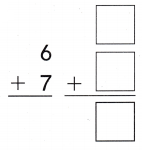Given,
By adding six with seven we get thirteen,
By changing the order of the addends, the sum will always be same,
By adding seven with six we get thirteen.

Question 3.Given,
By adding seven with five we get twelve,
In the same way, by changing the order of the addends we get the same sum,
By adding five with seven we get twelve,

Question 4.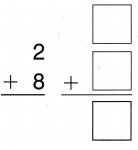Given,
By adding two with eight we get ten,
By changing the order of the addends, sum remains the same
By adding eight with two we get ten,

Question 5.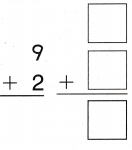Given,
By adding nine with two we get eleven,
In the same way, by changing the order of the addends we get the same sum,
By adding two with nine we get eleven,

Question 6.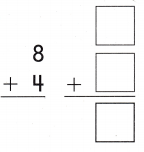Given,
By adding eight with four we get twelve,
By changing the order of addends, the sum remains the same,
By adding four with eight we get twelve,

Problem Solving

Question 7.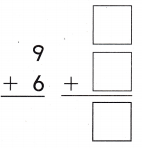Given,
By adding nine with six we get fifteen,
In the same way, by changing the order of the addends, the sum remains the same,
By adding six with nine we get fifteen,

Question 8.Given,
By adding seven with nine we get sixteen,
In the same way, we can change the order of the addends but the sum remains the same,
By adding nine with seven we get sixteen,

Question 9.Given,
By adding eight with three we get eleven,
In the same way, by changing the order of the addends we get the same sum
By adding three with eight we get eleven,

Question 10.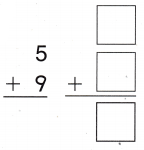Given,
By adding five with nine we get fourteen,
By changing the order of the addends we get the same sum
By adding nine with five we get fourteen,

Question 11.Given,
By adding four with five we get nine,
In the same way by changing the order of the addends we get the same sum,
By adding five with four we get nine,

Question 12.Given,
By adding eight with five we get thirteen,
In the same way we can change the order of the addends, but the sum remains the same,
By adding five with eight we get thirteen

Question 13.
H.O.T. Anna has two groups of pennies. She has 10 pennies in ail. When she changes the order of the addends, the addition sentence is the same. What sentence can Anna write?_________ = _________ + __________
Answer: 10 = 5 + 5
Given,
Anna has two groups of pennies has total of ten number of pennies,
By adding five with five we get ten,
In the same way, we can change the order of the pennies, the sum remains the same.

Question 14.
H.O.T. Multi-Step If Adam knows 4 + 7 = 11, what other addition fact does he know? Write the new fact in the box. Tell how Adam knows the new fact.
Given,
The addition of four with seven gets eleven,
In the same way, we can change the order of the addends but the sum remains the same,
By adding seven with four we get eleven,
We can change the order of the addends in any way, but the sum doesn’t change.
7 + 4 = 11

Question 15.
The number sentence 4 + 5 = 9 tells about q snails racing up trees. Which number sentence shows the addends in a different order?(A) 3 + 6 = 9
(B) 2 + 3 = 5
(C) 5 + 4 = 9
Given,
The number sentence as by adding four with five gives nine,
By changing the addends, we can get the same sum
So the order of the addends can be changed and the correct number sentence,
We get it by adding five with four we get nine.

Question 16.
Analyze Joey uses the number sentence 3 + 7 = 10 to tell about his toy trucks. What other number sentence tells about his trucks?(A) 6 + 4 = 10
(B) 7 + 3 = 10
(C) 4 + 3 = 7
Given,
The number sentence is three with seven we get ten,
In the same way, we can change the order of the addends and the sum remains same,
So by adding seven with three we get ten.

Question 17.
John wrote the number sentence 5 + 3 = 8. Which shows the same addends in a different order?
(A) 3 + 5 = 8
(B) 5 + 5 = 10
(C) 5 + 8 = 13
Given,
The number sentence is adding five with three to get eight,
By changing the order of the addends we can get the same sum,
So by adding three with five we get eight.

Question 18.
Texas Test Prep Which shows the same addends in a different order?
9 + 3 = 12
(A) 6 + 3 = 9
(B) 3 + 9 = 12
(C) 4 + 8 = 12
Given,
By adding nine with three we get twelve,
In the same way, by changing the order of the addends we get the same sum,
So by adding three with nine we get twelve.

TAKE HOME ACTIVITY • Ask your child to explain what happens to the sum when you change the order of the addends.
Example:
Explanation:
Here, by adding two with three we get the sum as five,
In the same way, we can add three with two while the sum remains the same.
We can take any kind of two different or the same addends and by adding them we get the sum,
In the same way we can change the order of the addends and still we get the same sum.

### Texas Go Math Grade 1 Lesson 12.1 Homework and Practice Answer Key

Question 1.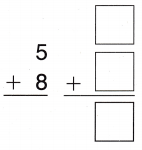Given,
By adding five with eight we get thirteen,
If we change the order of the addends we can see the sum remaining the same,
By changing the order of the addends and adding eight with five we get the sum as thirteen.

Question 2.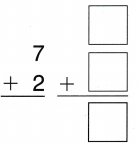Given,
By adding seven with two we get nine,
Even though by changing the order of the addends we can get the same sum,
By adding two with nine we get the sum as nine.

Question 3.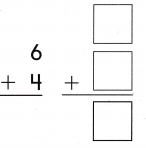Given,
By adding six with four we get the sum as ten,
In the same way, we change the order of the addends still we get the same sum,
So by changing and adding four with six we get the sum as ten.

Question 4.Given,
By adding nine with three we get twelve,
We can change the order of the addends, and get the same sum,
By adding three with nine we can get twelve as the sum.

Question 5.Given,
By adding seven with eight we get fifteen,
We can change the order of the addends, but we can get the same sum,
By adding eight with seven we get fifteen,
So the answer remains the same.

Question 6.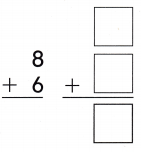Given,
By adding eight with six we get fourteen,
If we change the order of the addends, we can get the same sum,
By changing and adding six with eight we get the sum as fourteen.

Problem Solving

Question 7.
If Lorena knows that 6 + 9 = 15, what other addition fact does she know? Write the new fact in the box. Tell how Lorena knows the new fact.
Answer: 9 + 6 = 15
Explanation:
Given,
Lorena knows adding six with nine we get fifteen,
She can change the order of the addends to get the same sum and can make another addition fact
By changing the addends, we can add nine with six to get fifteen as the sum.

Lesson Check

Question 8.
Alice uses the number sentence 5 + 9 = 14 to tell about the number of laps she ran. Which number sentence shows the addends in a different order?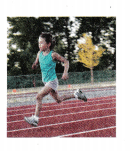(A) 5 + 8 = 14
(B) 9 + 5 = 14
(C) 4 + 5 = 9
Explanation:
Given,
Number sentence is adding of five with nine to get fourteen,
Even if we change the order of addends, we can get the same sum,
By adding nine with five we get fourteen without any change in the sum we can change the order of the addends to get a different addition fact.

Question 9.
The number sentence 3 + 6 = 9 tells about 9 pieces of fruit. What other number sentence tells about the same pieces of fruit?
(A) 3 + 2 = 5
(B) 4 + 4 = 8
(C) 6 + 3 = 9
Answer: The correct sentence is option – C
Explanation:
Given,
Number sentence is adding three with six to get nine as the sum,
In the same way, by changing the order of the addends we can get the same sum without any change it,
By adding six with nine we can get the sum which remains same with no changes even though there is a change in order of addends.

Question 10.
Multi-Step Jake writes an addition sentence. When he changes the order of the addends, the addition sentence is the same. Which addition sentence can Jake write?
(A) 3 + 5 = 8
(B) 8 + 8 = 16
(C) 5 + 6 = 11
Answer: All the above are correct.
Explanation:
Given,
As the given sentences all belongs to addition facts,
Even though changing the order of the addends the sum remains same,
So all the above number sentences can change the order of the addends but still the sum remains the same.

Question 11.
Which shows the same addends in a different order?
4 + 9 = 13
(A) 9 + 4 = 13
(B) 13 – 4 = 9
(C) 7 + 6 = 13